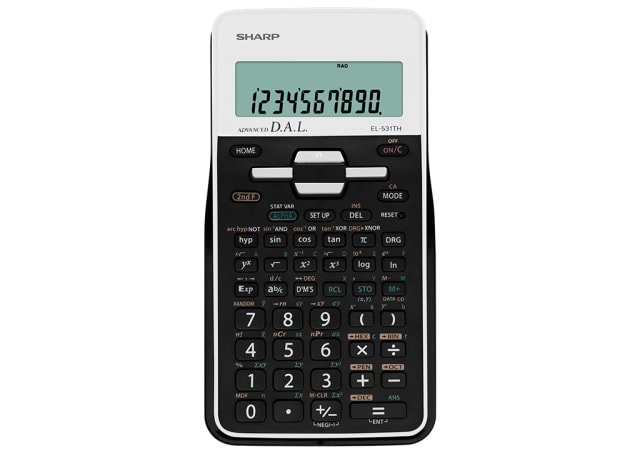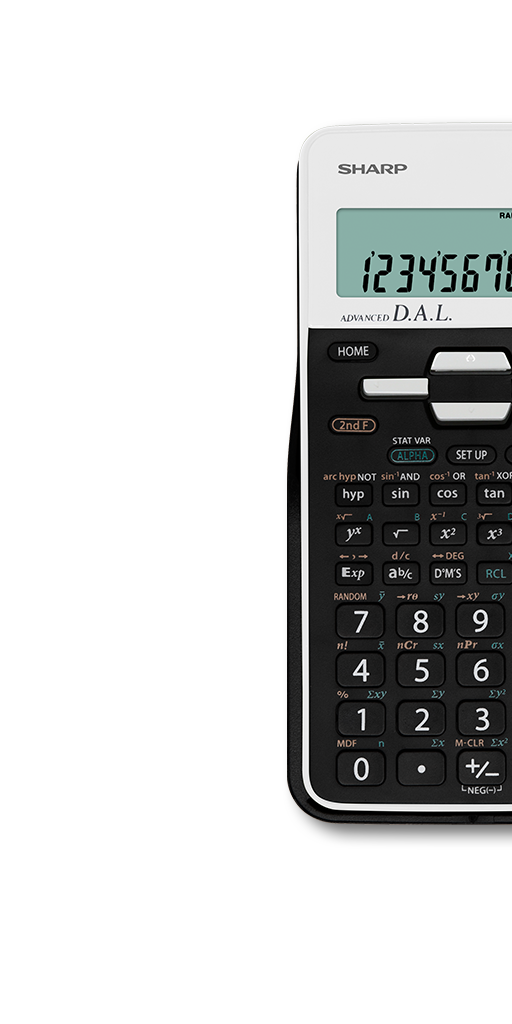EL531THBWH

# Scientific Calculator - White/ Black

• Recommended High School Model
• Approved by NSW Board of Studies
• Large, high resolution, 2 line LCD screen with upper 12 digit (5x7 - dot matrix)/lower 10 digit (mantissa) + 2 (exponent) digit
• Up to 273 scientific and statistical functions
• Battery operated (1xAAA)
POA## Scientific Calculator## LCD Display## Battery Operated## Protective Hard Cover• A scientific calculator designed for usage in science, engineering, and mathematics. Great for school or engineers.
• Two-line display showing equation entered on top line and answer on the bottom line allowing users to check both the equation and answer at the same time. User can amend the equation if needed
• Powered by 1 x AAA battery. Battery is secured with child-safe lock.
• Recall previous equations, storing up to 142 characters. 9 values can be stored in calculator, with 8 temporary memories and 1 independent memory. *Normal mode only
• Calculator is approved by the Board of Studies. Can be used in schools
• Store up to 273 scientific and statistical functions in calculator
• Hard cover to protect calculator from scratches and for easy storage. Great for students to store their calculators in their school bags
• "A portion of the equation is automatically memorised as a constant ( function Plus, Minus, Division, Multiplication) , thus omitting redundant input. E.g. 2 x 5 = 10 pressing = will multiply by 5 again
• This function is great for instances where you'll need to calculate the same equation with a different variable e.g. stocktake or estimating sales
• The square root of a number is a value that when multiplied by itself equals the original number. Great for jobs like architects, carpenters and engineers
• For easier reading of long, multi-digit numbers, "," or " ' " is inserted for each group of three digits. E.g. 10 thousand will displayed as 10,000
• Allows you to enter numbers as fractions
• Allow equations to be entered without having to key the answer from previous equation repeatedly
• To use the calculator for statistic calculations. Great for students or engineers
Calculator Types
Scientific Calculators
Display
2 Line LCD
Dimensions (WxHxD)
80 x 161 x 15 millimetre
Power Source
1 x AAA
Memory
9
Constant
Yes
Key Material
Plastic
Scientific & Stats function
273
Multi-line Playback
Yes
Chain Calculation
Yes
Fraction Key
Yes
Statistics mode
Yes
Hard Cover
Yes, slide-on